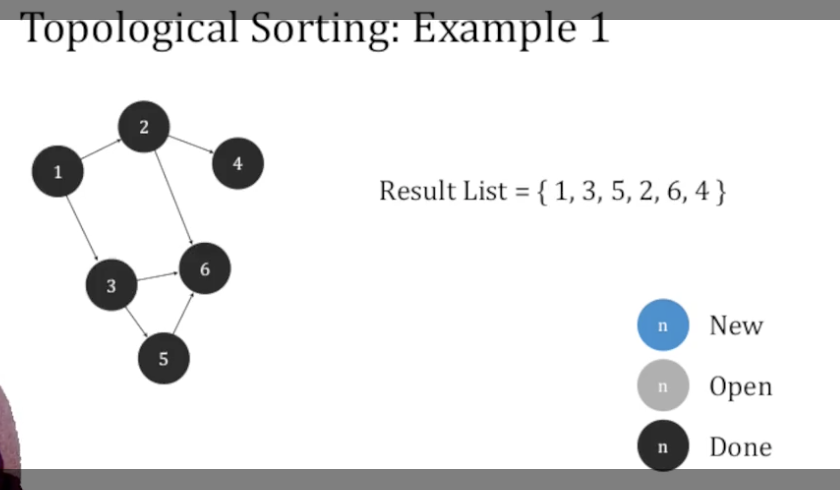# 数据结构与算法： 图遍历和拓扑排序

## Graph Traversal techniques and Topological Sorting

Posted by R1NG on February 11, 2022 Viewed Times

# 图遍历和拓扑排序

## 1. 图

(Graph) 由 节点集 $V$边集 $E$ 组成, 并满足 $E \subseteq V \times V$, 即任何边的顶点都位于节点集中.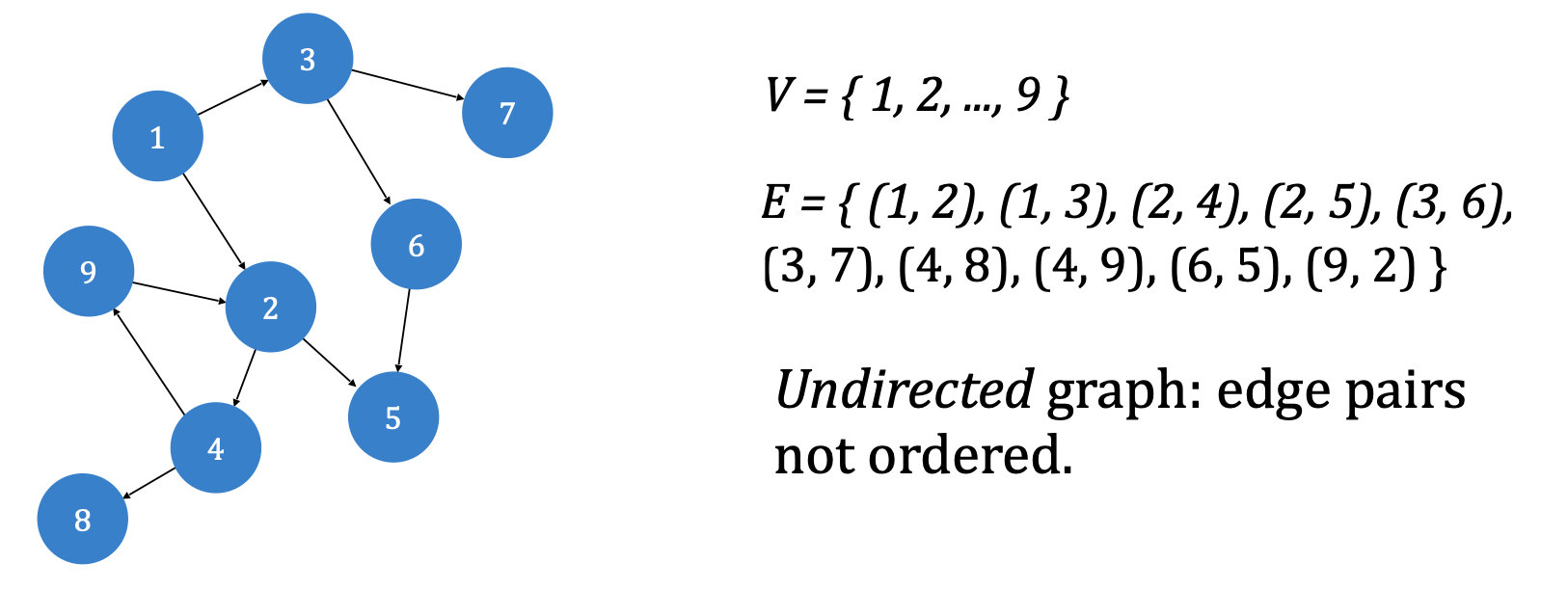$u_1\cdots u_n$1. 图中不存在任何平行的边, 即任何两条边的起始节点和终止节点都不完全相同.
2. 图中不存在任何起始节点和终止节点相同的边.

$V' \subseteq V, ~ E' \subseteq E.$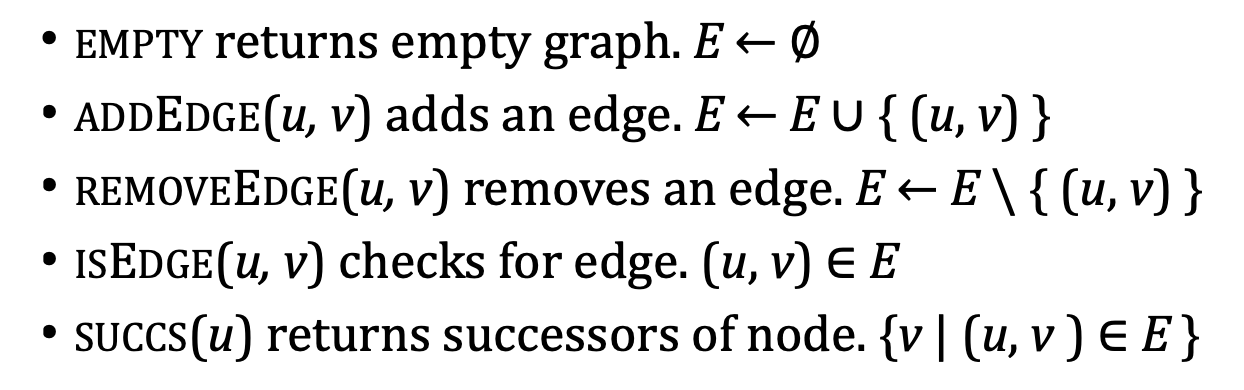### 1. 图的邻接列表实现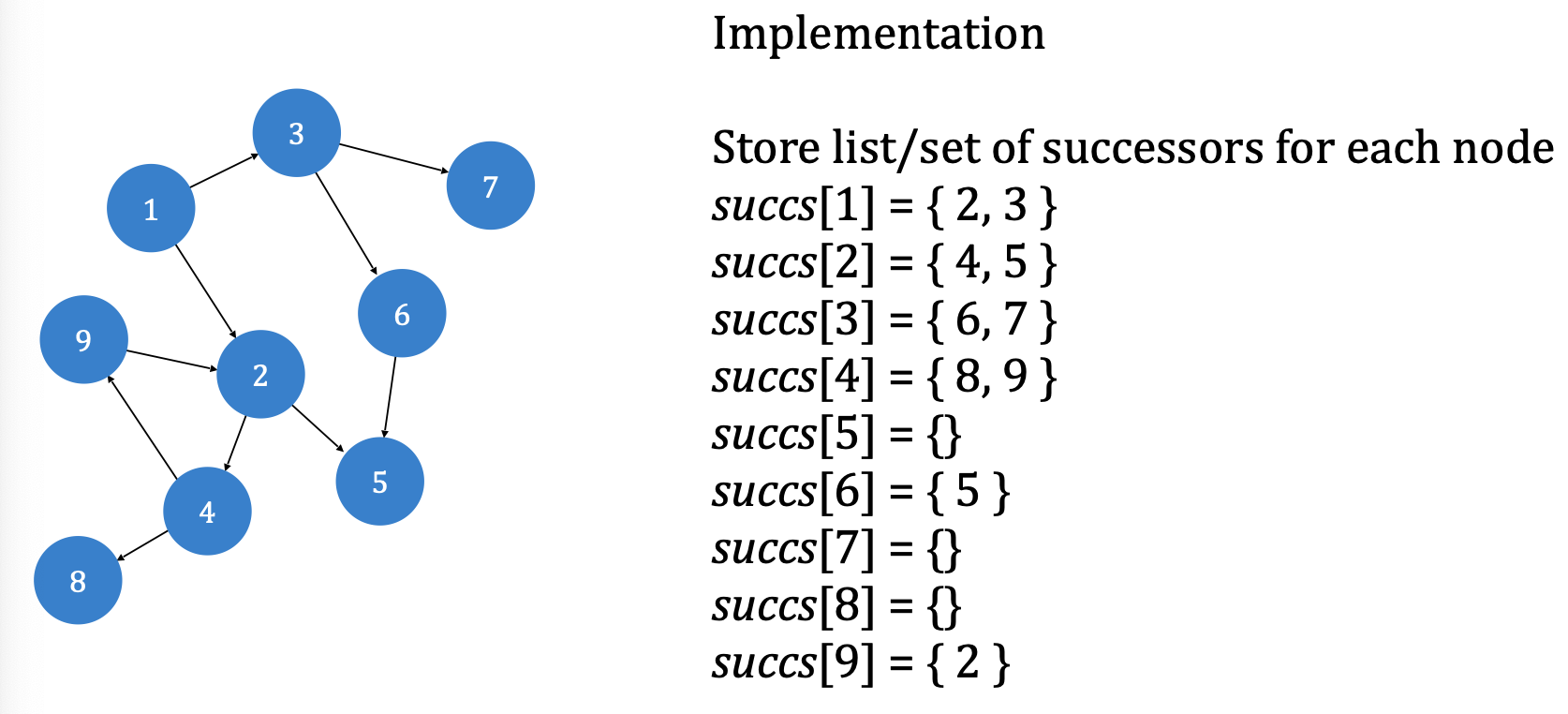$E = \{(u, v) \vert v \in \text{succ}(u)\}.$

$O(\vert V \vert + \vert E \vert),$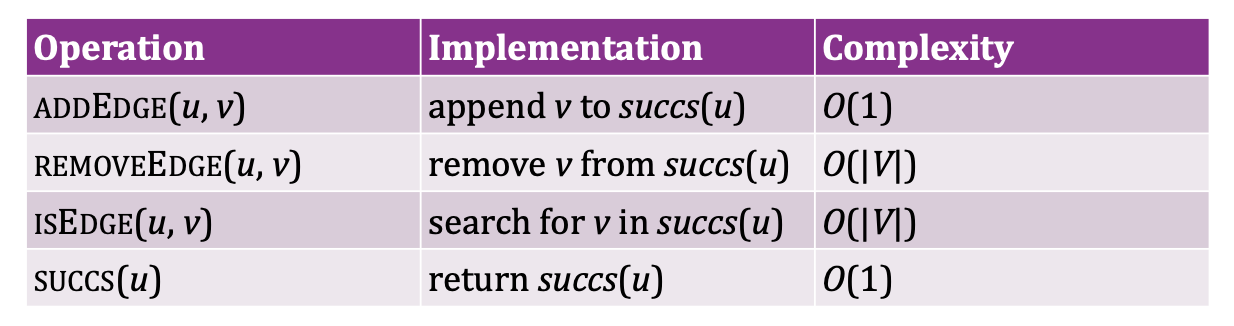### 2. 图的邻接矩阵实现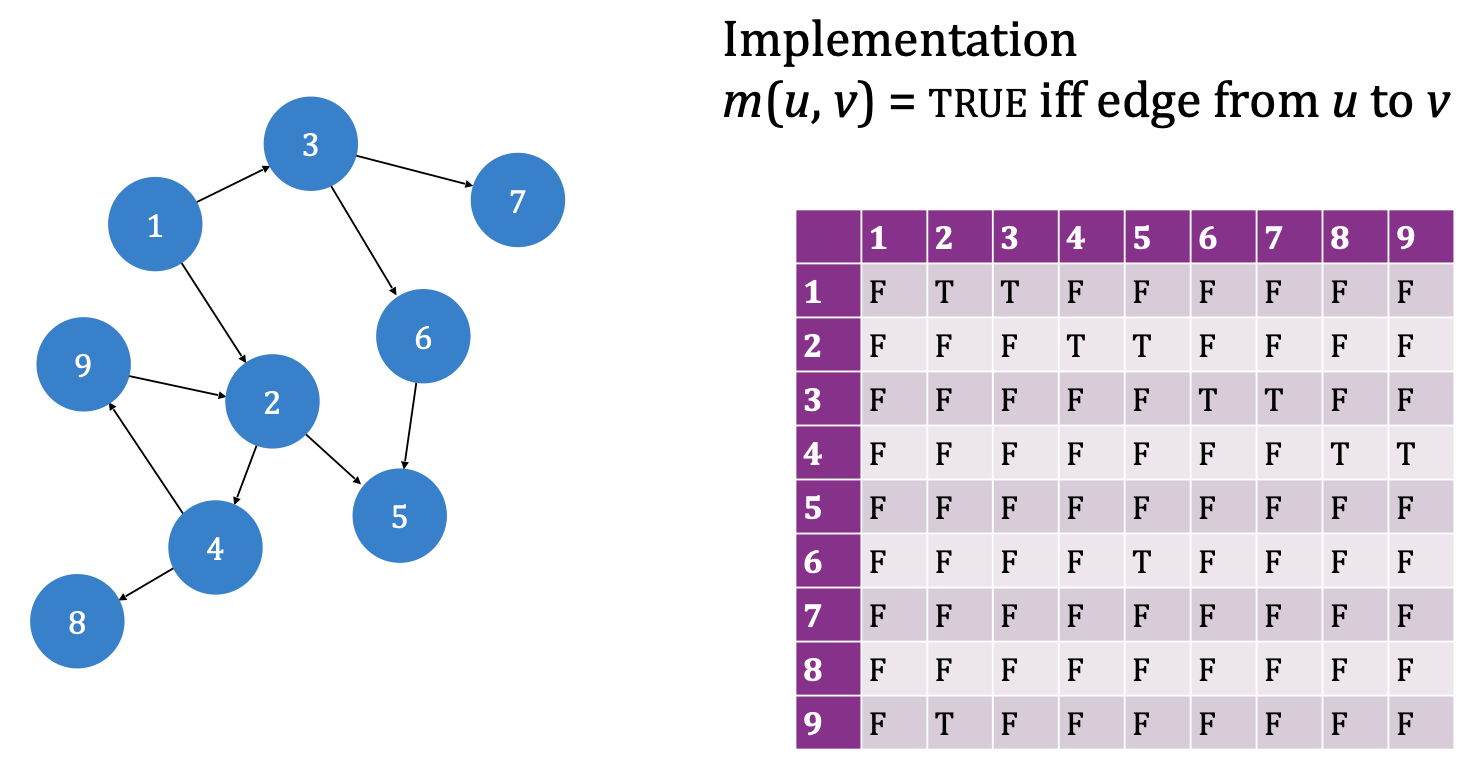$O(\vert V \vert^2),$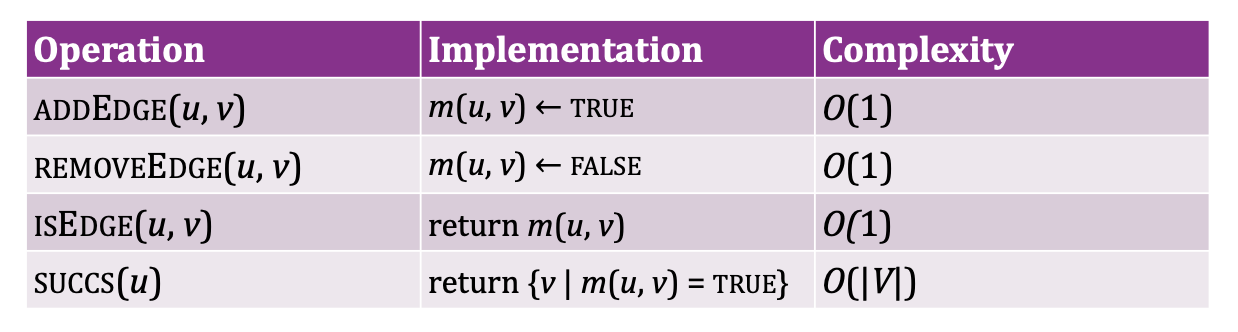## 2. 图遍历

### 2.1 通用算法

1. $F$: 已经遍历过的边.
2. $D$: 已经发现但尚未检查以它为出发点的边的节点.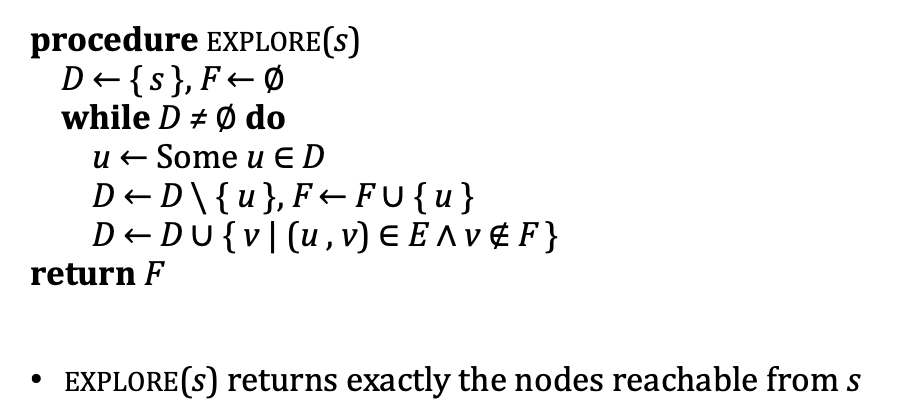### 2.2 深度优先与广度优先算法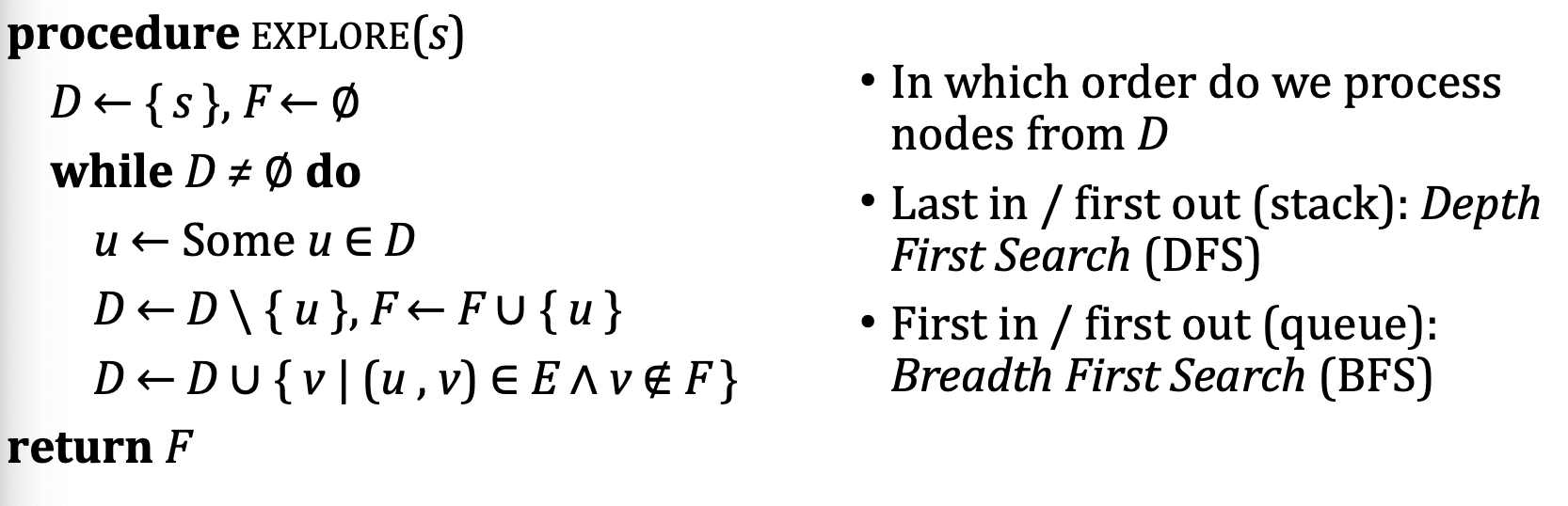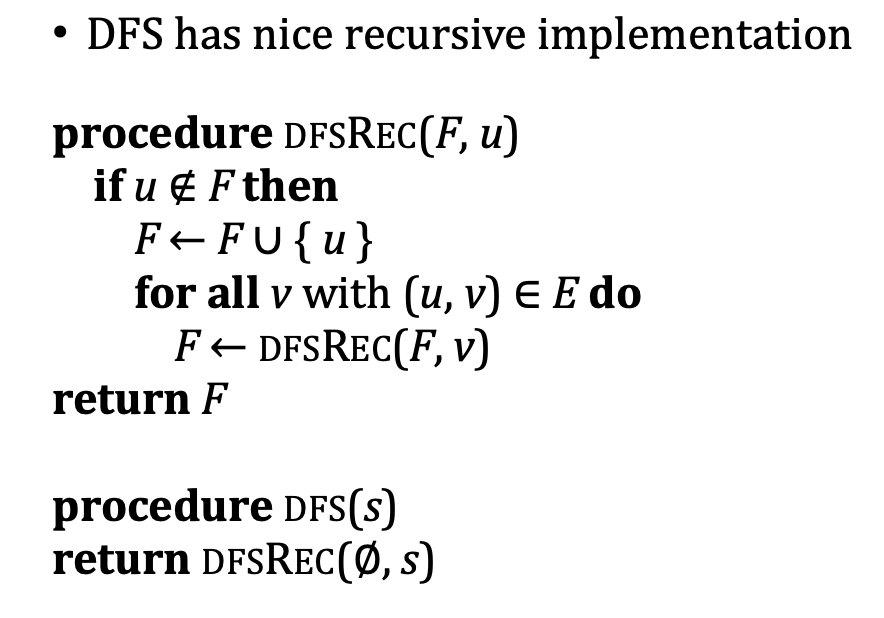1. 求解深度優先和廣度優先時, 務必不要想當然, 而是 嚴格構造 Discovered 棧/隊列 和 Finished 列表, 一步步地進行推導!
2. 對於深度優先算法, 若從 Discovered 中彈出某個節點, 檢索其子節點中有 Discovered 中元素時, 須將 Discovered 中對於元素刪除, 然後再按照順序插入.

## 3. 拓扑排序

1. 存在一系列任务, 需要以合适的方式排序从而生成一个可供规划的任务执行顺序.
2. 这一系列任务之间存在依赖关系, 即: 某些任务是其他一些任务的前束条件, 只有在执行它后才能进一步执行其他的任务.
3. 任务之间的关系可被建模为有向图: 任务之间的依赖关系可用有向边表示.

1. 首先将图中的所有节点标记为 $N$ (New).
2. 在检索图时, 若首次遇到了某个节点 $n$, 则将其标记为 $O$ (Open).
3. 因此, 在遍历过程中只要发现遇到了任何标记为 $O$ 的节点, 则这条路径一定是圈.
4. 只有在遍历完它的全部子节点后, 才将其标记为已完成遍历的节点 $D$ (Done).